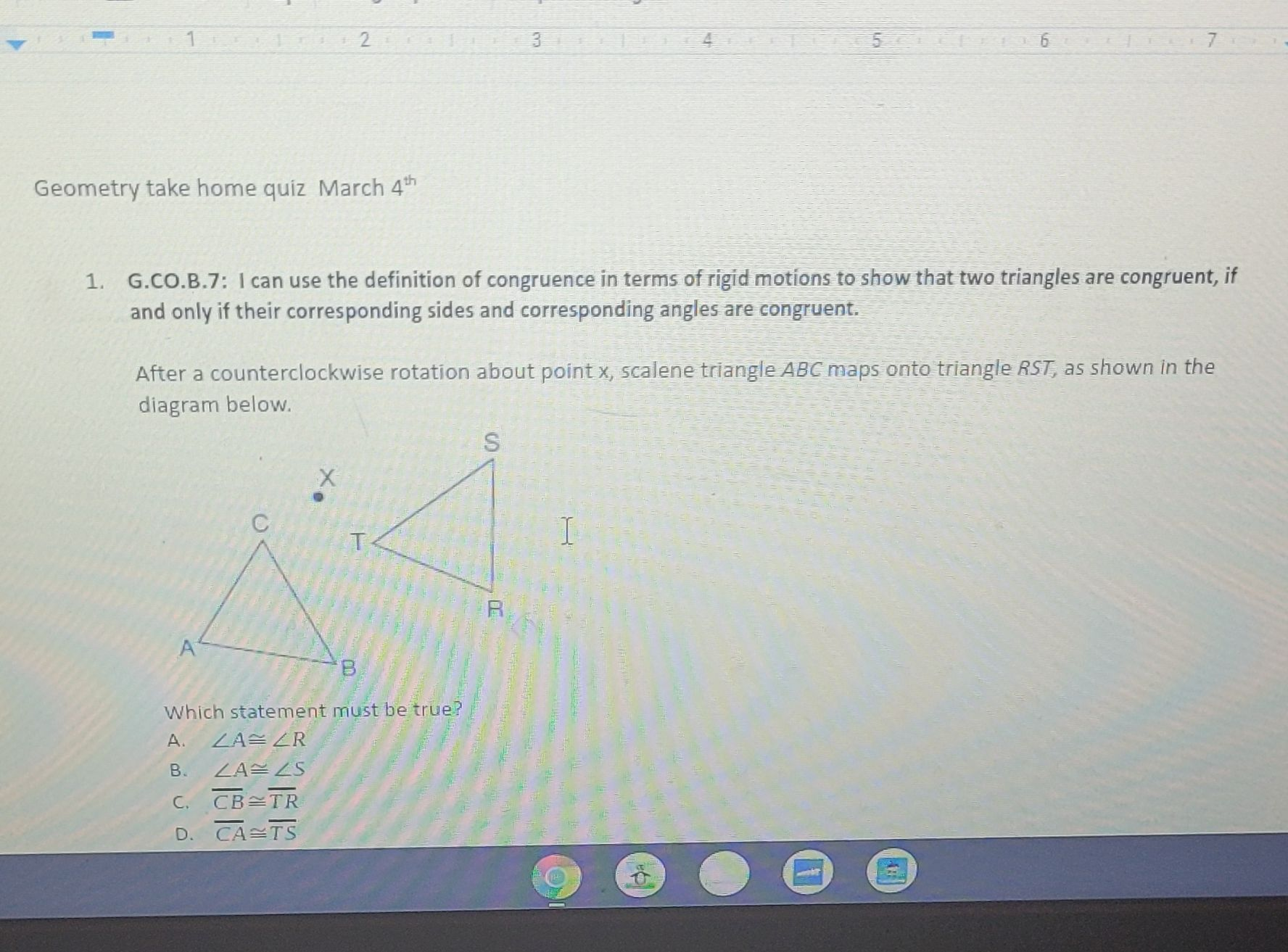### Still have math questions?

Trigonometry
Question1. G.CO.B.7: I can use the definition of congruence in terms of rigid motions to show that two triangles are congruent, if and only if their corresponding sides and corresponding angles are congruent. After a counterclockwise rotation about point x, scalene triangle $$A B C$$ maps onto triangle $$R S T$$ , as shown in the diagram below. Which statement must be true? A. $$\angle A \cong \angle R$$

B. $$\angle A \cong \angle S$$

C. $$\overline { C B } \cong \overline { T R }$$

D. $$\overline { C A } \cong \overline { T S }$$